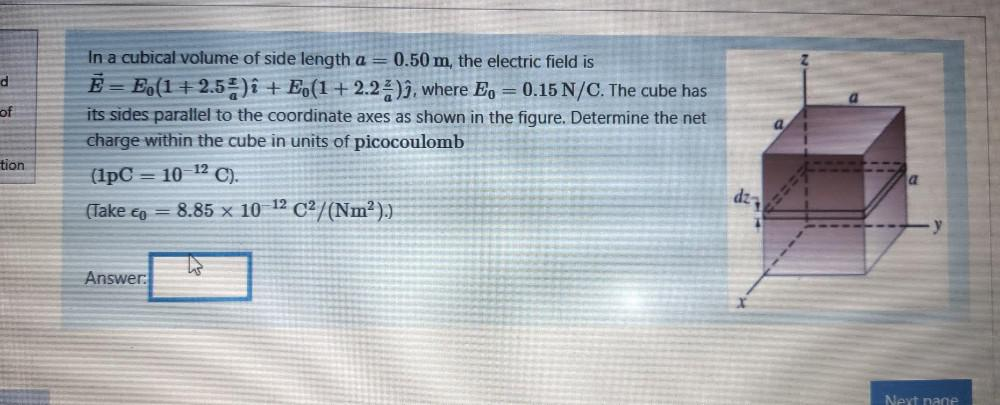Question:

# d of In a cubical volume of side length a = 0.50 m, the electric field is Ē= E.(1 +2.5%)i + E (1+2.2%)ì, where E, = 0.15 N/C. Thd of In a cubical volume of side length a = 0.50 m, the electric field is Ē= E.(1 +2.5%)i + E (1+2.2%)ì, where E, = 0.15 N/C. The cube has its sides parallel to the coordinate axes as shown in the figure. Determine the net charge within the cube in units of picocoulomb (1pC = 10-12 C). (Take cp = 8.85 x 10-12 C2/(Nm²).) (co ) tion 017 Answer: Next page